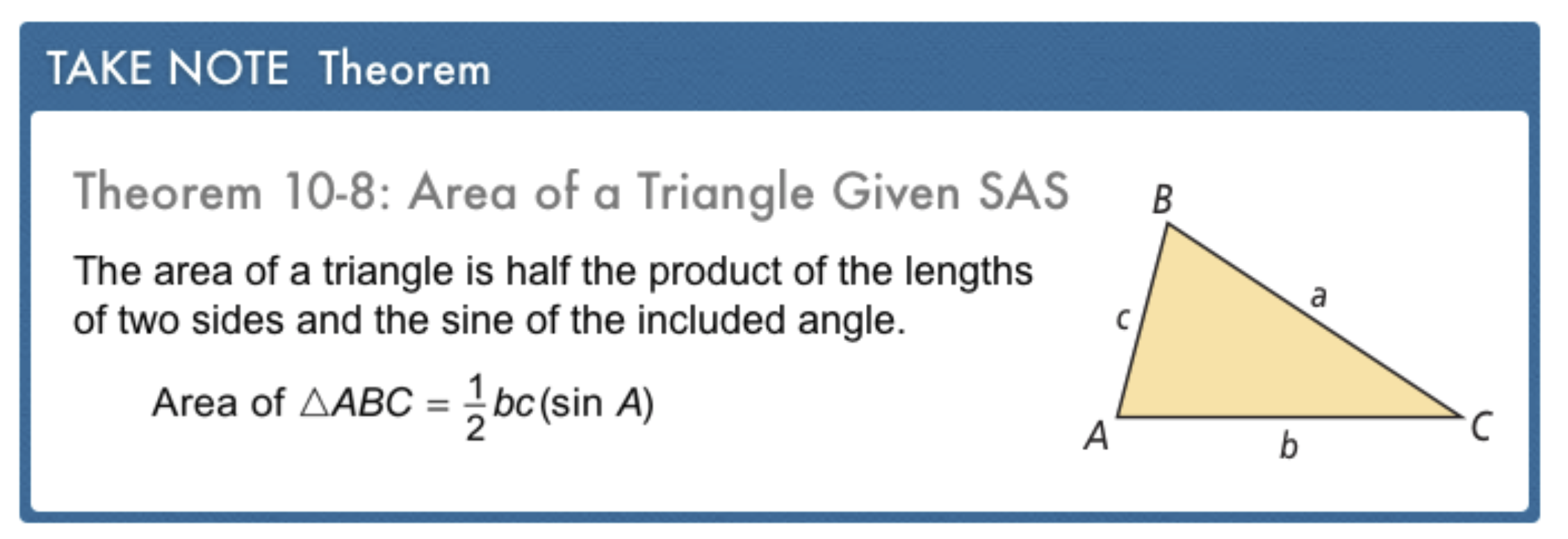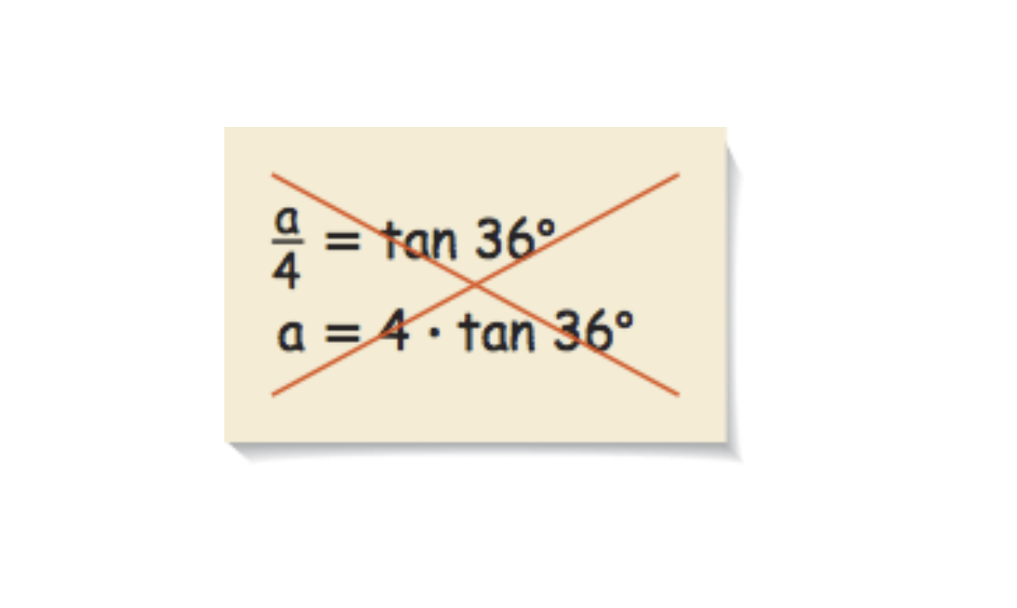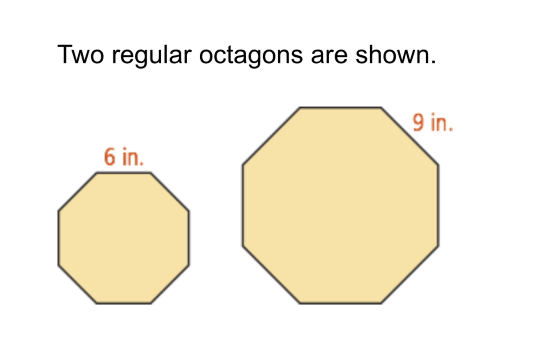Geometry 10-5 Trigonometry and Area
starstarstarstarstarstarstarstarstarstar
by Matthew Richardson
| 22 Questions
Note from the author:
A complete formative lesson with embedded slideshow, mini lecture screencasts, checks for understanding, practice items, mixed review, and reflection. I create these assignments to supplement each lesson of Pearson's Common Core Edition Algebra 1, Algebra 2, and Geometry courses. See also mathquest.net and twitter.com/mathquestEDU.
The outlined content above was added from outside of Formative.
The outlined content above was added from outside of Formative.1
2
1
10 pts
Solve It! The pennant shown is in the shape of an isosceles triangle. The measure of the vertex angle is 20 degrees. What is the area of the pennant? Hint: Consider using right triangle trigonometry. Round to the nearest square inch and enter your response in this format: 981 square inches
2
10 pts
Solve It! Explain how you found your answer to the previous item.
The outlined content above was added from outside of Formative.3
3
10 pts
Problem 1 Got It?
A
B
C
D
The outlined content above was added from outside of Formative.
4
10 pts
Problem 2 Got It? A tabletop has the shape of a regular decagon with a radius of 9.5 in. What is the area of the tabletop to the nearest square inch? Enter your response in this format: 981 square inches
5
10 pts
Problem 2 Got It? Reasoning: Suppose the radius of a regular polygon is doubled. How does the area of the polygon change? Explain.
The area is doubled. The doubling of the radius indicates a doubling in the apothem and area as well.
The area is tripled. The doubling of the radius indicates a doubling in the apothem and area.
The area is quadrupled. The doubling of the radius indicates a doubling in the apothem and area.The outlined content above was added from outside of Formative.6
6
10 pts
Problem 3 Got It?
A
B
C
D7
7
10 pts
A
B
C
D8
8
10 pts
A
B
C
D9
9
10 pts
A
B
C
D11
11
10 pts
Error Analysis: Your classmate needs to find the area of a regular pentagon with 8-cm sides. To find the apothem, he sets up and solves a trigonometric ratio. What error did he make? Explain.12
13
12
10 pts
Review Lesson 10-4: What is the scale factor of the smaller octagon to the larger octagon?

13
10 pts
Review Lesson 10-4: The area of the larger octagon is 391.1 in². What is the area of the smaller octagon to the nearest square inch? Enter your response in this format: 39 square inches
The outlined content above was added from outside of Formative.14
15
14
10 pts
Review Lesson 8-5: Use the Law of Sines to find the value of x. Round to the nearest hundredth. Enter your response in this format: 21.30 m
15
10 pts
Review Lesson 8-5: Use the Law of Sines to find the value of y. Round to the nearest hundredth. Enter your response in this format: 21.30 m
16
10 pts
Review Lesson 1-8: Sort the items in ascending order (least on top) based on the value of the number that fills in each blank.
1. A circle with radius 6 has diameter __?__.
2. A circle with radius 7 has diameter __?__.
3. A circle with diameter 15 has radius __?__.17
10 pts
Vocabulary Review: Fill in the blank to complete the sentence.

Area is the number of __?__ units needed to cover a given surface.
cubic
square
18
10 pts
Vocabulary Review: What is the formula for the area of a triangle?
A = bh
A = ½bh
A = ½h(b₁ + b₂)
A = ½d₁d₂19
5 pts
Use Your Vocabulary: Write only the word trigonometry or trigonometric to complete the sentence. The sine, cosine, and tangent ratios are __?__ ratios.
20
5 pts
Use Your Vocabulary: Write only the word trigonometry or trigonometric to complete the sentence. This year I am studying __?__ in math.
21
10 pts
Use Your Vocabulary: Match each ratio of side lengths from a right triangle with the trigonometric ratio it represents.

• cosine
• sine
• tangent
22
10 pts
Reflection: Math Success